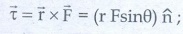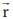Home | | Physics 11th std | Short Questions and Answer

## Chapter: 11th Physics : UNIT 5 : Motion of System of Particles and Rigid Bodies

Physics : Motion of System of Particles and Rigid Bodies : Book Back Important Questions, Answers, Solutions : Short Questions and Answer

Motion of System of Particles and Rigid Bodies (Physics)

1. Define center of mass.

The center of mass of a body is defined as a point where the entire mass of the body appears to be concentrated.

2. Find out the center of mass for the given geometrical structures.

a) Equilateral triangle b) Cylinder c) Square3. Define torque and mention its unit.

Torque is defined as the moment of the external applied force about a point or aixs of rotation.unit is Nm.

4. What are the conditions in which force can not produce torque?

● Torque is zero whenandare parallel. (θ = 0°)

● Torque is zero whenandare anti parallel. (θ = 180°)

● Torque is zero if the force acts at the reference point is= 0,= 0.

5. Give any two examples of torque in day-to-day life.

● Opening and closing of a door about the hinges

● Turning of a nut using a wrench.

6. What is the relation between torque and angular momentum?

τ = Iα

τ = I [dω / dt]

τ = Id(Iω) / dt

L = Iω

τ = I [dL/dt]

7. What is equilibrium?

A rigid body is said to be in mechanical equilibrium when both its linear momentum and angular momentum remains constant.

8. How do you distinguish between stable and unstable equilibrium?Stable equilibrium

1. The body tries to come back to equilibrium

2. The center of mass of the body shifts slightly higher

3. Potential energy, of the body is minimum and it increases if disturbed.

4. Linear momentum and angular momentum are zero.

Unstable equilibrium

1. The body cannot come back to equilibrium

2. The center of mass of the body shifts slightly lower

3. Potential energy of the body is not minimum and it decreases if disturbed.

4. Linear momentum and angular momentum are zero.

9. Define couple.

A pair of forces which are equal in magnitude but opposite in direction and separated by a perpendicular distance so that their lines of action do not coincide that causes a turning effect is called a couple.

10. State principle of moments.

The principle of moments states that, in equilibrium, the total sum of the anti-clock wise moment is equal to the total sum of the clockwise moment.

11. Define center of gravity.

The centre of gravity of a body is the point of which the entire weight of the body acts irrespective of the position and orientation of the body.

12. Mention any two physical significance of moment of inertia

i) It is a measure of how difficult it is to rotate a particular body about a given axis.

ii) It is not an invariable quantity.

iii) It depends not only on the mass of the body, but also on the mass which is distributed around the axis of rotation.

13. What is radius of gyration?

It is the perpendicular distance from the axis of rotation to an equivalent point mass, which would have the same mass as well as the same moment of inertia of the object.

14. State conservation of angular momentum.

When no external torque acts on the body the net angular momentum of a rotating rigid body remains constant.

15. What are the rotational equivalents for the physical quantities, (i) mass and (ii) force?

i) Mass equivalent for moment of inertia.

I = m r2

ii) Force equivalent for torque, τ = Iα

16. What is the condition for pure rolling?

● As the point of contact is at momentary rest.

● Resultant velocity is zero.

● VTRANS = VROT

● VCM = Rω

17. What is the difference between sliding and slipping?Sliding

1. VCM > Rω

2. VTRANS > VROT

3. This happens when sudden break is applied in a moving vehicle

4. It is also referred as forward clipping

Slipping

1. VCM < Rω

2. VTRANS < VROT

3. This happens when we suddenly start the vehicle from rest.

4. It is sometimes referred as backward slipping.

Tags : Motion of System of Particles and Rigid Bodies | Physics , 11th Physics : UNIT 5 : Motion of System of Particles and Rigid Bodies
Study Material, Lecturing Notes, Assignment, Reference, Wiki description explanation, brief detail
11th Physics : UNIT 5 : Motion of System of Particles and Rigid Bodies : Short Questions and Answer | Motion of System of Particles and Rigid Bodies | Physics